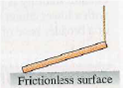# Problem: The uniform rod in Figure 15 has a weight of 14.0 N. What is the magnitude of the normal force exerted on the rod by the surface?A. 7NB. 14 NC. 20 ND. 28N

###### FREE Expert Solution

We'll solve this problem using the sum of forces for a system in equilibrium.

For a system in equilibrium:

$\overline{){\mathbf{\Sigma }}{{\mathbf{F}}}_{\mathbf{u}\mathbf{p}}{\mathbf{=}}{\mathbf{\Sigma }}{{\mathbf{F}}}_{\mathbf{d}\mathbf{o}\mathbf{w}\mathbf{n}}}$

94% (231 ratings)###### Problem Details

The uniform rod in Figure 15 has a weight of 14.0 N. What is the magnitude of the normal force exerted on the rod by the surface?
A. 7N
B. 14 N
C. 20 N
D. 28N